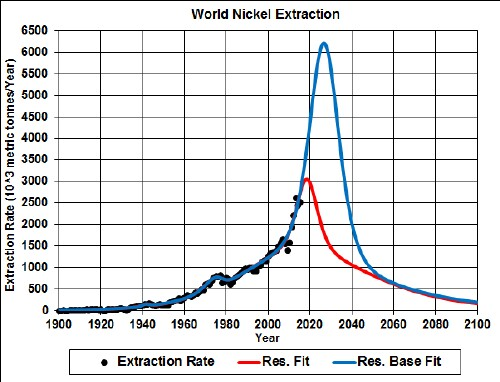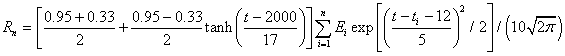# Nickel Depletion Including Recycling

Nickel is an important metal. It is used to make modern batteries: Nickel-Cadium and Nickel-Metal-Hydride. The graph below shows the nickel extraction data for the world and two Verhulst function fits to the data in order to extrapolate into the future.Nickel extraction rate for the world and two Verhulst functions fits to the data.

The red curve is a fit with total amount to be extracted of 135 x 106 tonnes, which incorporates the latest reserves estimate, and the blue curve is fit with total amount to be extracted of 210 x 106 tonnes, which incorporates the latest reserves-base estimate.

It appears that world-nickel extraction will peak before 2025.

Taking an average extraction curve of the two fits, the crossover point at ~2023 when the amount extracted is equal to the amount left to be extracted is shown here:## Recycling

Assume that:

• The nickel extraction curve is the average of the two curves given above.
• Recycling of nickel follows a hyperbolic tangent curve from 30% to 90% recycling with a break point of year 1950 and width 50 years.
• The recycling is delayed by a Gaussian curve peaking at a delay of 25 years and a width of 10 years.

The effective nickel available for making items after the first ten recycling cycles is shown in the following graph, along with the effective nickel available for each cycle:## Recycling

Nickel, especially the nickel used in batteries, is recycled to a high degree. Assume that:

• The nickel-extraction curve is the average of the two curves given above.
• Recycling of nickel follows a hyperbolic tangent curve from 30% to 95% recycling with a break point of year 2000 and width 17 years.
• The recycling is delayed by a Gaussian curve peaking at a delay of 12 years and a width of 5 years.

The effective nickel available for making items after the first ten recycling cycles is shown in the following graph, along with the effective nickel available for each cycle:The equation for a recyling cycle is,

where Ei is the amount available from the previous cycle. Here is an example of the Excel coding:

{=((\$J\$2+\$I\$2)/2+((\$J\$2-\$I\$2)/2)*TANH((A27-\$K\$2)/\$L\$2))*SUM(\$I\$27:I27*(EXP(-1*((A27-\$A\$27:A27-\$N\$2)/\$O\$2)^2/2))/\$O\$2/SQRT(2*PI()))} (The curly bracket surrounding the term makes it into an array; it must be entered by holding down the SHIFT & CTRL keys while pressing the ENTER key.)

Of course, the recycling could be extended to more cycles, skewing the curve further into the future. However, the peak and fall off after it will not change because further cycles are essentally zero in that time region.

Thus, under the assumptions given above, the effective amount of nickel available for making items peaks at about 2060 and falls off rapidly after that. Humans will have taken concentrated nickel deposits and scattered them across the surface of the earth.

The Excel spreadsheet is set up to make it easy to calculate with different recycling assumptions.

## Nickel Per Capita

Dividing the recycling curve above by the projected world population (asymptote ~1010), one gets:Minerals Depletion